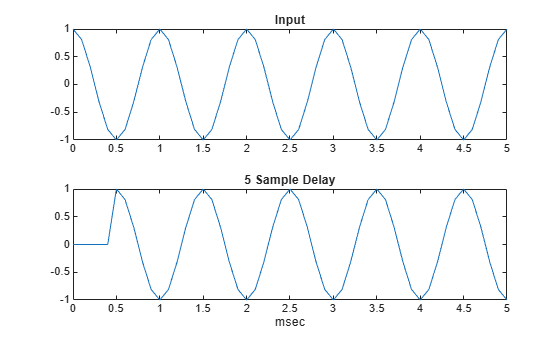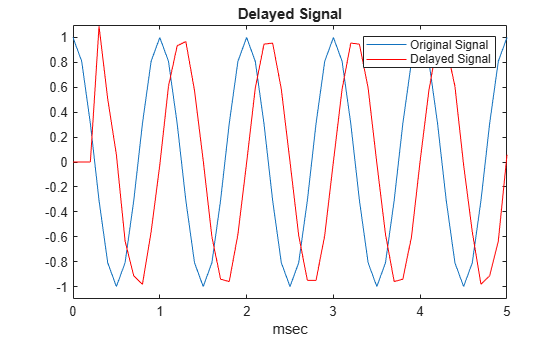Documentation

# delayseq

Delay or advance sequence

## Syntax

```shifted_data = delayseq(data,DELAY) shifted_data = delayseq(data,DELAY,Fs) ```

## Description

`shifted_data = delayseq(data,DELAY)` delays or advances the input `data` by `DELAY` samples. Negative values of `DELAY` advance `data`, while positive values delay `data`. Noninteger values of `DELAY` represent fractional delays or advances. In this case, the function interpolates. How the `delayseq` function operates on the columns of `data` depends on the dimensions of `data` and `DELAY`:

• If `DELAY` is a scalar, the function applies that shift to each column of `data`.

• If `DELAY` is a vector whose length equals the number of columns of `data`, the function shifts each column by the corresponding vector entry.

• If `DELAY` is a vector and `data` has one column, the function shifts `data` by each entry in `DELAY` independently. The number of columns in `shifted_data` is the vector length of `DELAY`. The kth column of `shifted_data` is the result of shifting `data` by `DELAY(k)`.

`shifted_data = delayseq(data,DELAY,Fs)` specifies `DELAY` in seconds. `Fs` is the sampling frequency of `data`. If `DELAY` is not divisible by the reciprocal of the sampling frequency, `delayseq` interpolates to implement a fractional delay or advance of `data`.

## Input Arguments

 `data` Vector or matrix of real or complex data. This argument supports single and double precision. `DELAY` Amount by which to delay or advance the input. If you specify the optional `Fs` argument, `DELAY` is in seconds; otherwise, `DELAY` is in samples. This argument supports single and double precision. `Fs` Sampling frequency of the data in hertz. If you specify this argument, the function assumes `DELAY` is in seconds. This argument supports single and double precision. Default: `1`

## Output Arguments

 `shifted_data` Result of delaying or advancing the data. `shifted_data` has the same number of rows as `data`, with appropriate truncations or zero padding.

## Examples

collapse all

Delay a cosine signal an integral number of samples.

```fs = 1.0e4; t = 0:1/fs:0.005; signal = cos(2*pi*1000*t)';```

Set the delay to 0.5 ms or 5 samples.

`shifted_signal = delayseq(signal,0.5e-3,fs);`

Plot the original and delayed signals.

```subplot(2,1,1) plot(t.*1000,signal) title('Input') subplot(2,1,2) plot(t.*1000,shifted_signal) title('0.5-msec delay') xlabel('msec')```Delay a 1 kHz cosine signal by a fractional number of samples. Assume a sampling rate of 10 kHz.

```fs = 1e4; t = 0:1/fs:0.005; signal = cos(2*pi*1000*t)';```

Set the delay to 0.25 ms or 2.5 samples.

`delayed_signal = delayseq(signal,0.25e-3,fs);`

Plot the original signal (blue) and delayed (red) signals.

```plot(t.*1000,signal) title('Delayed Signal') hold on plot(t.*1000,delayed_signal,'r') axis([0 5 -1.1 1.1]) xlabel('msec') legend('Original Signal','Delayed Signal') hold off```The values of the delayed signal amplitudes differ from the original signal due to the interpolation used in implementing the fractional delay.

## See Also

#### Hybrid Beamforming for Massive MIMO Phased Array Systems

Download the white paper